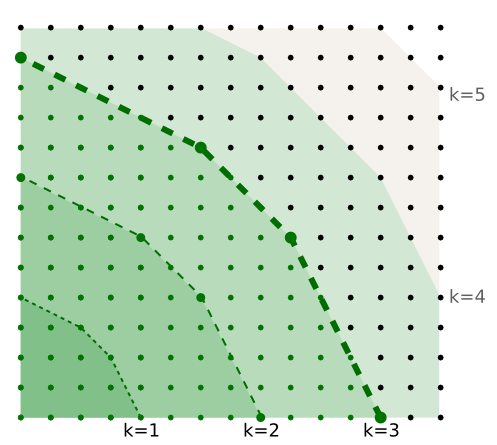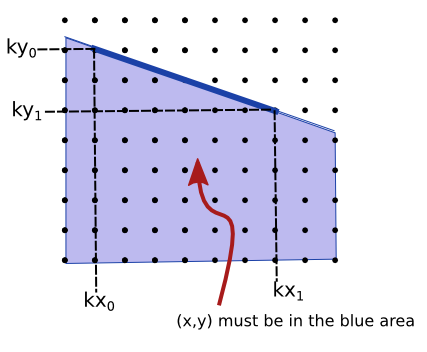## Friday, July 05, 2013

### Editorial for TopCoder Open round 3B ToastJumping

I know that this is very late, but I was finally able to understand this problem and the medium.

## ToastJumping

Problem statement

You jump between lattice points, starting at (0,0), the length of a jump cannot exceed sqrt(d), what is the minimum number of jumps needed to reach (x,y), there are up to 50 queries of this kind.

Each test case involves a number of queries. Since every argument in the queries can vary, including d[i], we should just treat each query as a unique case independently. This approach has the consequence that the time limit of 2 seconds is effectively divided by 50. We should make sure not to take more than 40 milliseconds in a single query.

## Points reachable in a single jump

Let us move on assuming a single query: Minimum number of jumps from (0,0) to (x,y), if the length of the jumps is at most sqrt(d).

The set of points reachable using at most one jump is interesting. For small values of d, it is a bit trivial, so let us try d=9, thus sqrt(d) = 3. The distance must be at least 3, the reachable points are all those lattice points inside a circle of radius 3:## Points reachable in two jumps

It might take a while to finally notice how this set of points looks like. For each of the points reachable in one jump, find the points that can be reached in one jump starting at that point. This means that there are other circles.The property that will allow us to solve the problem lies hidden in the above image but it is not too easy to notice it without a different perspective.

## Polygon of reachable points

The points reachable in a single jump are those inside a circle, but since they are all lattice points, they do not really make a circle shape. The set of reachable points is merely a convex polygon:The lattice points that coincide with this polygon are important. Because those extreme moves are possible in one step, we can also assume the other moves inside the convex polygon they generate are possible too. Let us draw those moves as vectors:When we try the points reachable in two jumps, something interesting happens:The polygon of points reachable in two jumps is actually a scaled version of the original polygon. For each coordinate (x,y) of perimeter of the original polygon, the new polygon will have a point (2x,2y).

## Generalizing

This property is true for any value of k and for any value of d, if the points of the polygon that contains points reachable in 1 jump are (x_i,y_i), the points of the polygon that contains points reachable in k jumps will be: (k * x_i, k * y_i).

For a demonstration, start by saying that point (x_i,y_i) is reachable in one jump. If we repeat the same jump k times, then (k * x_i, k* y_i) is also reachable in k jumps. The space of reachable points is convex, so we can assume that all points inside the convex hull of points (k * x_i, k * y_i) will be reachable in k jumps. This shows that the points inside the polygon are reachable. We also need to show that the points outside the polygon aren't reachable. Let us use a less formal approach for this: The points in the perimeter of the polygon are the the reachable points that are the furthest away from the origin, in order to move the furthest distance from the origin in a direction, it is most efficient to use that same direction in each jump.

## Solution

The solution is then to find the smallest k such that the convex hull (k * x_i, k * y_i) contains point (x,y). Implementing the solution is another story.

The polygon will be symmetric in each quadraint, so we can just assume that (x,y) belongs to the first quadraint (x,y >= 0), if that wasn't the case, we can just use their absolute values thanks to symmetry. We will need to care only about a quarter of the polygon. For sqrt(d)=4, k<=5:We only need to generate the points (x_i, y_i) of the first polygon. In order to do that, we can try, for each px, the maximum y such that (px,py) is reachable in one jump. We need to repeat for each value px from 0 to sqrt(d). In order to find the value py, we can just assume it has to be less than or equal to the value for the previous px (As it is the first quadraint, the slopes in the polygon are decreasing). This ensures we only try each candidate py from sqrt(d) to 0 exactly once. Thus finding the points (px,py) will take O(sqrt(d)).

After generating those points, they will not necessarily be a convex polygon. They will look like this:This polygon isn't convex. This can be solved by taking the Convex Hull of all the points (px,py) we find. Since we will already find each point in clockwise order, we do not need to sort the points, so it is possible to do the Graham Scan in O(sqrt(d)) time.

### Minimum k

After we find the points (px,py) of the polygon of points reachable in one jump, we still need to find the minimum k. Unfortunately, an approach such as using a binary search for k may not be efficient enough.

What we can do is take each segment (k*px_i, k*py_i), (k*px_(i+1), k*py_(i+1)) as a constraint for the point. The point must lie inside the polygon, which means that the point should be below the line formed by the segment. For simplicity, we will rename the points of the segment (x_0,y_0), (x_1,y_1).From the line equation we have:

y <= ky_0 - (x - kx_0) * (ky_0 - ky_1) / (kx_1 - kx_0)
y <= ky_0 - (x - kx_0) * (y_0 - y_1) / (x_1 - x_0)

Let D = (y_0 - y_1) / (x_1 - x_0):

y <= ky_0 - (x - kx_0) * D
y <= k * (y_0 + x_0 * D) - Dx
k >= (y + Dx) / (y_0 + Dx_0)
...
k >= ( x * (y_0 - y_1) + y * (x_1 - x_0) ) / ( x_1 * (y_0 - y_1) + y_1 * (x_1 - x_0) )

Since k must be an integer, the minimum k greater than or equal to that expression is the ceiling function of that expression.

Each segment determines a lower bound k, k must be at least that value. The maximum of those lower bounds is the minimum valid k. There are O(sqrt(d)) segments thus the overall algorithm is still O(sqrt(d)).

### Code

public long area2(long x1, long y1, long x2, long y2, long x3, long y3) {    // Returns 2 * area of the triangle using cross product.    // If the result is negative, the points are in anti-clockwise order.    return (x2 - x1) * (y3 - y1) - (x3 - x1) * (y2 - y1);}long px[], py[];int makePolygon(long d){    // Returns the convex hull of the points in quadraint  that are    // reachable in one jump.    final int MAX_SQD = 31623;    int sz = 0;    px = new long[MAX_SQD+1];    py = new long[MAX_SQD+1];    long y = MAX_SQD;    for (long x=0; ; x++){        //decrease y until it is inside the circle / is 0:        while(y > 0 && x*x + y*y > d) {            y--;        }        if (x*x + y*y > d) {            // x is outside the circle:            break;        }        //Direction of the segments in the polygon should be clockwise:        // If the new point makes an anti-clockwise direction with the previous        // point (aread2 > 0), then we need to remove the previous point to        // keep the polygon convex and so and so for each of the previous points.        // We can also remove the previous point if they are colinear        // (area2 == 0), but it is  not necessary for the solution.        while(sz >= 2 && area2(px[sz-2], py[sz-2], px[sz-1], py[sz-1], x, y) >= 0) {            // (If the triangle area is 0, the points are colinear)            sz--; //remove last point        }        // [Basically a simplified version of Graham's scan, we already find the        // points in clockwise order.]                //Add the point:        px[sz] = x; py[sz] = y; sz++;    }    // Make sure to close the polygon...    if (py[sz-1] != 0) {        px[sz] = px[sz-1];        py[sz] = 0;        sz++;    }    return sz;}// Solve for each x[i],y[i],d[i]:public long minJumpsSingle(long x, long y, long d){        x = Math.abs(x);    y = Math.abs(y);    int sz = makePolygon(d);        long k = 0;    for (int i=0; i<sz-1; i++) {        long dy = py[i] - py[i+1], dx = px[i+1] - px[i];        long p = x * dy + y * dx;        long q = px[i] * dy + py[i] * dx;        // ceil(p/q) is the minimum k such that the point (x,y) will        // be below line that visits points:        //    (k*px[i], k*py[i]) ,  (k*px[i+1], k*py[i+1])        k = Math.max(k, (p + q - 1) / q); // Do ceil(p/q) without floats.    }    return k;}